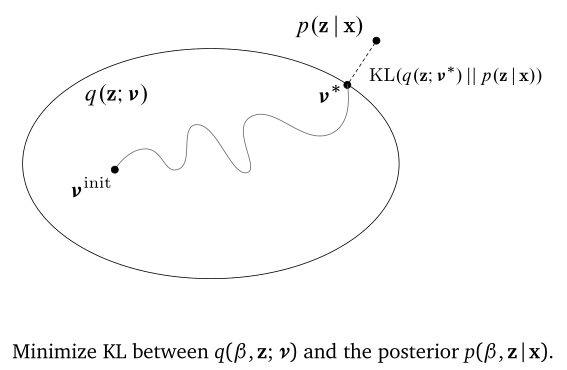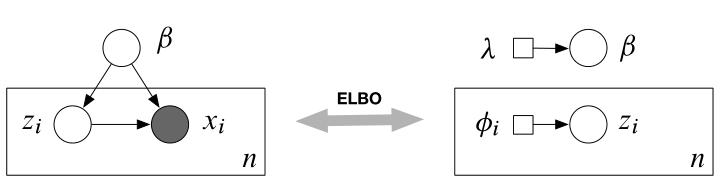## Variational Inference

• $\eta$ the natural parameter
• $t(x)$ the sufficient statistics
• $a(\eta)$ the log normalizer
• $h(x)$ the base density### Mean-filed VI### Recipe

1. Start with a model
$p(\mathbf{z}, \mathbf{x})$
2. 选择合适的变分分布
$q(\mathbf{z} ; \nu)$
3. 根据两种拆分方式写出 ELBO，如
$\mathscr{L}(\nu)=\mathbb{E}_{q(\mathbf{z} ; \nu)}[\log p(\mathbf{x}, \mathbf{z})-\log q(\mathbf{z} ; \nu)]$
4. 积分得到 ELBO
Example: $\mathscr{L}(\nu)=x \nu^{2}+\log \nu$
5. 关于变分参数求梯度，优化 ELBO
Example: $\nabla_{\nu} \mathscr{L}(\nu)=2 x \nu+\frac{1}{\nu}$
$nu_{t+1}=\nu_{t}+\rho_{t} \nabla_{\nu} \mathscr{L}$

## Black box VI

Define

Black Box Criteria

• sample from $q(\beta, \mathbf{z})$
• evaluate $q(\beta, \mathbf{z})$ or function of $q$
• evaluate $\log p(\beta, \mathbf{z}, \mathbf{x})$

• Score gradients
• Reparameterization gradients

### Score Function Gradients

See score function, it is called likelihood ratio or REINFORCE gradient.

• Sampling from $q(\mathbf{z})$
• Evaluating $\nabla_{\nu} \log q(\mathbf{z} ; \nu)$
• Evaluating $\log p(\mathbf{x}, \mathbf{z})$ and $\log q(\mathbf{z})$